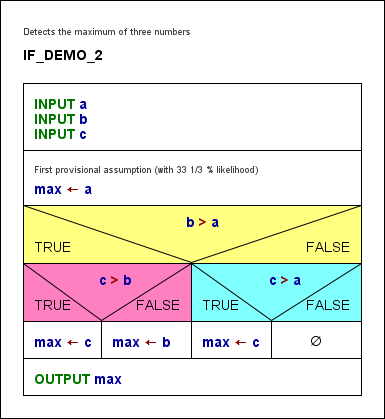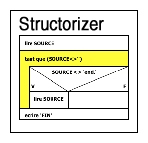S T R U C T O R I Z E R - User Guide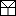Elements > IF statement
 An element of type IF statement represents an alternative in the control flow. It is used at a decision point where the algorithm must take one of two different ways. The IF statement comprises the condition and both emerging paths. It is important to understand, however, that both branches join together again at the bottom of the element, i.e. after having passed the selected branch (whichever of them it is), the control flow will continue below the IF element in either case. The text of the IF element is to contain the logical condition. It may be represented by any Boolean expression, i.e. an expression that evaluates either to true or to false. If the value computes to true then the left branch will be taken, otherwise the right branch. Please note:The labels for the "TRUE" and "FALSE" branch in the graphical presentation can be modified in Structure Preferences (menu item "Preferences › Structures"). But let us explain it with a simple example: imagine you have the task to find out whether an input value is greater than zero. This obviously requires first an input instruction for a variable, say A, and then a decision — you will have to compare the value of the variable with 0 and generate a different text output in both cases. This simple algorithm might look like in the screenshot below, where the entire IF statement including the branches is marked yellow (note that if you select an IF statement then only its "head", i.e. the upper rectangle consisting of the three triangles containing the condition and the branch labels will be highlighted, see step 5 below):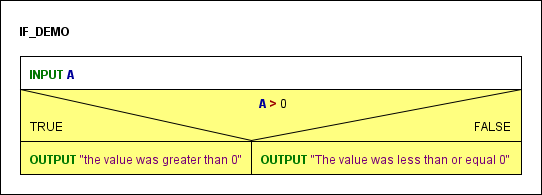If the condition comes true then the OUTPUT instruction in the left-hand branch (labelled TRUE) will be executed, otherwise the one in the right-hand branch (labelled FALSE). Now, how do you add an IF statement to your diagram? Assume the program is already named and the input instruction has already been placed (see Instruction): 1. Select the element where you want to append an IF statement, then click on the IF statement symbol in the toolbar (or press the accelerator key, or use the "Diagram" or context menu):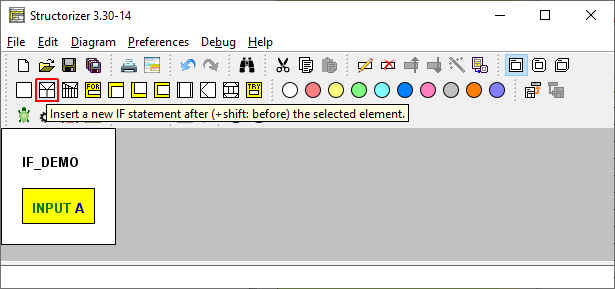2. Insert the condition When the element editor opens then the text field will contain some default string (which is configurable in the Structure Preferences). Replace this default string by the actual condition. This may be a comparison (via operators = or ==, <> or !=, <, >, <=, >=), a variable with Boolean content, or several conditions combined by logical operators. Accepted logical operators are and (also &&), or (also ||), not (also !), and xor (also ^), where the latter stands for exclusive or (P xor Q is true if exactly one of the conditions P and Q is true). The logical operators may also be written with upper-case letters (AND, OR, XOR, NOT). In our case the condition is A > 0: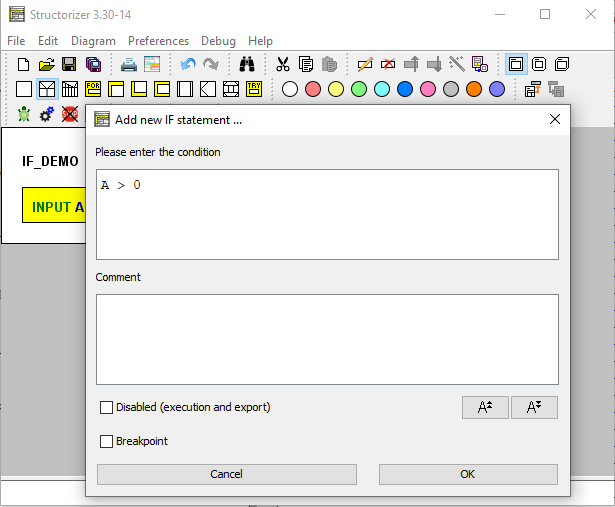If one of the two branches is meant to remain empty (i.e. you are just going to create a "conditioned instruction") then formulate the condition in such a way that the non-empty branch is the "TRUE" branch, which is always on the left-hand side. (If you accidently did otherwise you may simply have the branches swapped and the condition negated by means of transmutation.) 3. Add the elements to be executed depending on the condition, e.g. Instructions, to the respective branches (see Diagram/Add elements) by selecting the (empty) branch and pressing the toolbar button for the intended element type:Fill in the element texts according to the algorithm you have in mind (see above). 4. If you want to have more than one instruction executed in a branch then simply add them after or before the first one (i.e., make sure to have selected an element of the branch when inserting or appending another one):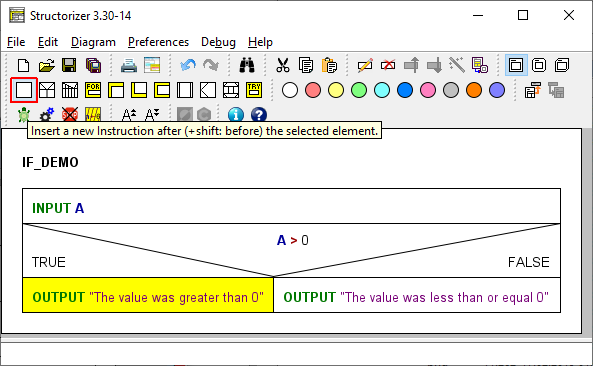5. If you want to add further elements after the entire IF statement (remember that, no matter which branch was chosen and executed, the control flow will continue with the element following to the IF statement) you must select its head first: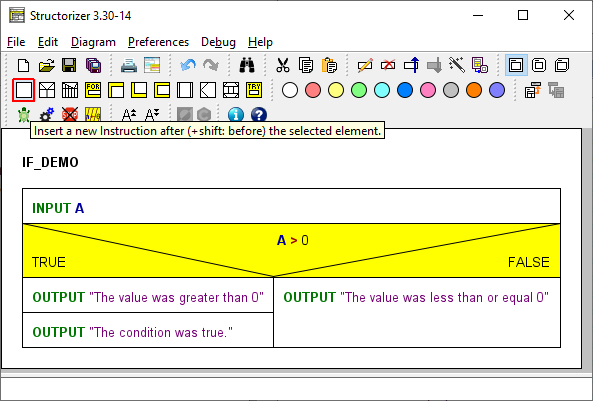6. After the insertion of an unconditioned OUTPUT instruction beneath the IF statement (note that neither the INPUT instruction at top nor the — now selected — OUTPUT instruction are part of the IF element), the algorithm would look like this: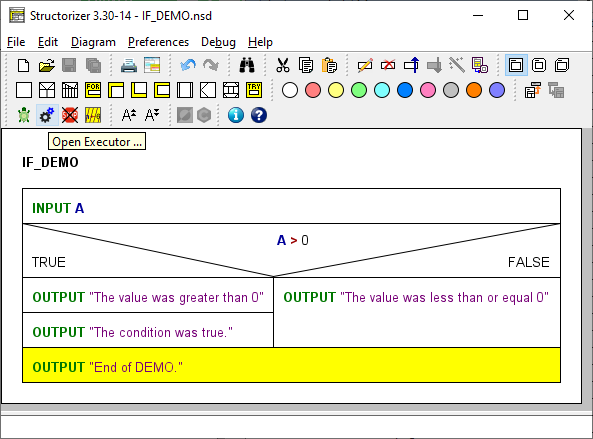Download Demo Alternatives (IF elements) may of course be nested (each IF branch may be a sequence of arbitrary elements, not only Instruction elements):